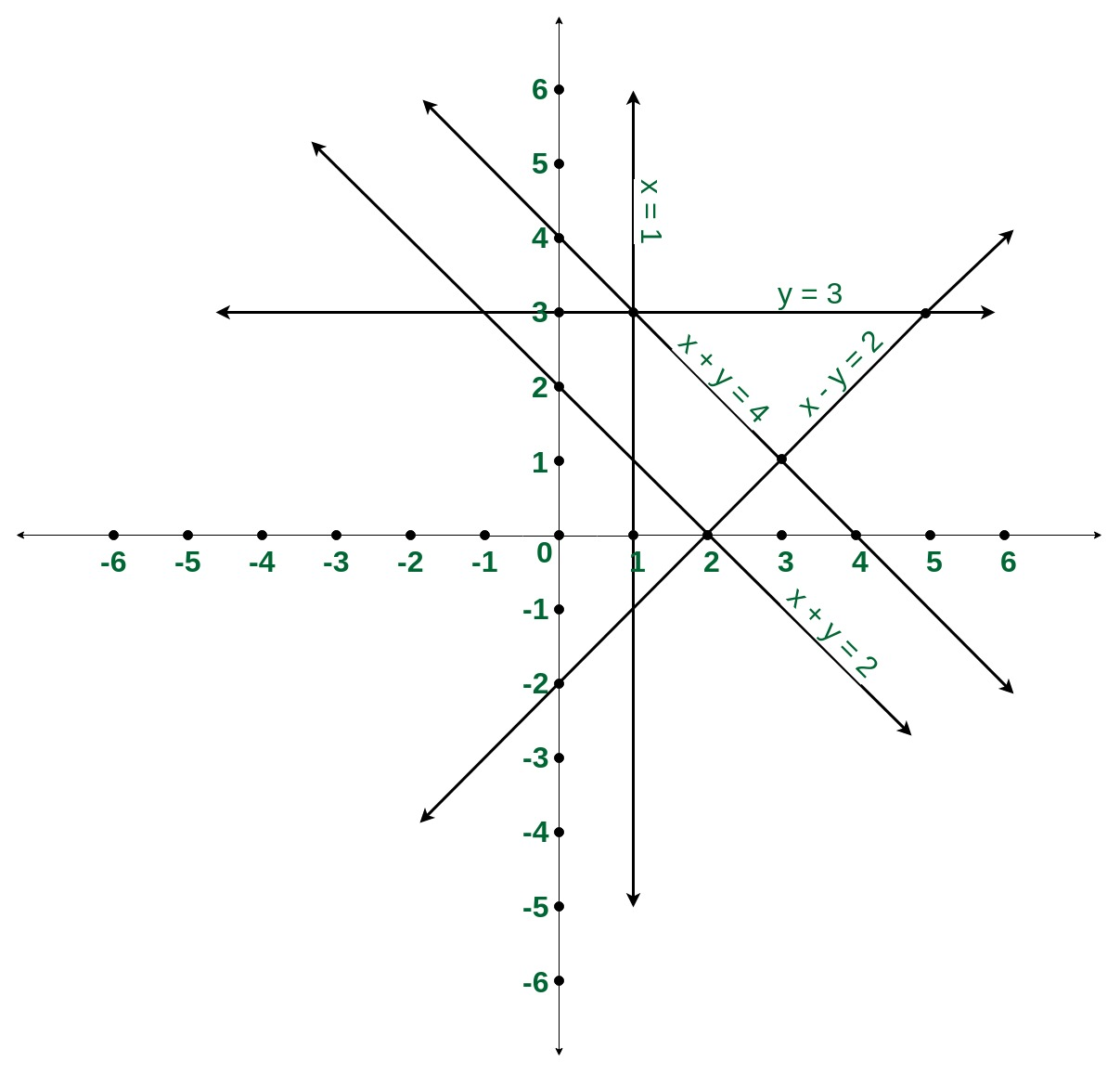# Count number of pairs of lines intersecting at a Point

Given N lines are in the form a*x + b*y = c (a>0 or a==0 & b>0). Find the number of pairs of lines intersecting at a point.

Examples:

Input: N=5
x + y = 2
x + y = 4
x = 1
x – y = 2
y = 3
Output: 9Input: N=2
x + 2y = 2
x + 2y = 4
Output: 0

## Recommended: Please try your approach on {IDE} first, before moving on to the solution.

Approach:

• Parallel lines never intersect so a method is needed to exclude parallel lines for each line.
• The slope of a line can be represented as pair(a, b). Construct a map with key as slope and value as a set with c as entries in it so that it has an account of the parallel lines.
• Iterate over the lines add them to the map and maintain a variable Tot which counts the total number of lines till now.
• Now for each line update the Tot variable then add Tot to the answer and subtract the number of parallel lines to that line including itself.

Below is the implementation of the above approach:

 `// C++ implementation to calculate ` `// pair of intersecting lines ` ` `  `#include ` `using` `namespace` `std; ` `  `  `// Function to return the number  ` `// of intersecting pair of lines ` `void` `numberOfPairs(``int` `a[],``int` `b[],``int` `c[],``int` `N){ ` ` `  `    ``int` `count = 0, Tot = 0; ` `  `  `    ``// Construct a map of slope and  ` `    ``// corresponding c value ` `    ``map, set<``int``> > LineMap; ` `  `  `    ``// iterate over each line ` `    ``for` `(``int` `i = 0; i < N; i++) { ` ` `  `        ``// Slope can be represented ` `        ``// as pair(a, b) ` `        ``pair<``int``, ``int``> Slope = ` `                     ``make_pair(a[i], b[i]); ` `  `  `        ``// Checking if the line does  ` `        ``// not already exist ` `        ``if` `(!LineMap[Slope].count(c[i])){ ` `            ``// maintaining a count ` `            ``// of total lines ` `            ``Tot++; ` `            ``LineMap[Slope].insert(c[i]); ` `  `  `            ``// subtracting the count of ` `            ``// parallel lines including itself ` `            ``count += Tot -  ` `                    ``LineMap[Slope].size(); ` `        ``} ` `    ``} ` `  `  `    ``cout << count << endl; ` `} ` ` `  `// Driver code ` `int` `main() ` `{ ` `    ``// A line can be represented as ax+by=c ` `    ``// such  that (a>0 || (a==0 & b>0) )  ` `    ``// a and b are  already in there lowest ` `    ``// form i.e gcd(a, b)=1 ` `    ``int` `N = 5; ` `    ``int` `a[] = { 1, 1, 1, 1, 0 }; ` `    ``int` `b[] = { 1, 1, 0, -1, 1 }; ` `    ``int` `c[] = { 2, 4, 1, 2, 3 }; ` `    `  `    ``numberOfPairs(a,b,c,N); ` `  `  `    ``return` `0; ` `} `

Output:

```9
```

Time Complexity:My Personal Notes arrow_drop_upCheck out this Author's contributed articles.

If you like GeeksforGeeks and would like to contribute, you can also write an article using contribute.geeksforgeeks.org or mail your article to contribute@geeksforgeeks.org. See your article appearing on the GeeksforGeeks main page and help other Geeks.

Please Improve this article if you find anything incorrect by clicking on the "Improve Article" button below.

Article Tags :
Practice Tags :

Be the First to upvote.

Please write to us at contribute@geeksforgeeks.org to report any issue with the above content.